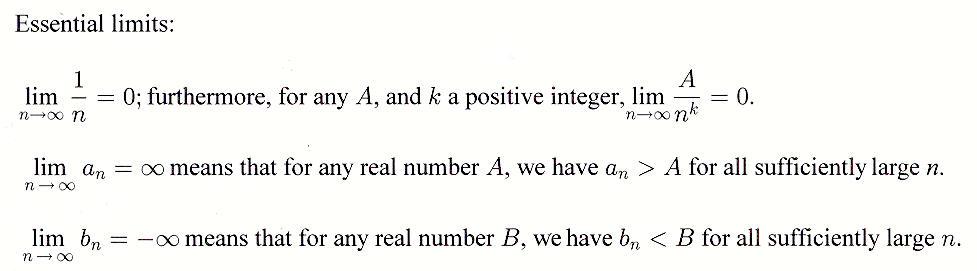## 18.2 Outline

1. Introduction
1. sequence
1. general term
2. successor
3. predecessor
2. notation
2. The limit of a sequence
3. Limits to infinity
1. converges
2. limit of the sequence
3. diverges
4. limit of 1/n to infinity
4. Infinite limits

## 18.2 Essential IdeasSee the whole entry

## 10.1 Outline

1. Exponential equation
1. definition
2. evaluate exponentials
2. Definition of logarithm
1. notation, logarithm, and argument
2. common logarithm
3. natural logarithm
3. Evaluating logarithms
1. evaluate
2. using calculators
3. change of base
4. exact solution
4. Exponential equations
1. three types
1. base 10
2. base e
3. base b

2. micometer

See the whole entry